# Advanced VLOOKUP in Excel: multiple, double, nested

These examples will teach you how to Vlookup multiple criteria, return a specific instance or all matches, do dynamic Vlookup in multiple sheets, and more.

It is the second part of the series that will help you harness the power of Excel VLOOKUP. The examples imply that you know how this function works. If not, it stands to reason to start with the basic uses of VLOOKUP in Excel.

Before moving further, let me briefly remind you the syntax:

VLOOKUP(lookup_value, table_array, col_index_num, [range_lookup])

Now that everyone is on the same page, let's take a closer look at the advanced VLOOKUP formula examples:

## How to Vlookup multiple criteria

The Excel VLOOKUP function is really helpful when it comes to searching across a database for a certain value. However, it lacks an important feature - its syntax allows for just one lookup value. But what if you want to look up with several conditions? There are a few different solutions for you to choose from.

### Formula 1. VLOOKUP with two criteria

Suppose you have a list of orders and want to find the quantity based on 2 criteria, Customer name and Product. A complicating factor is that each customer ordered multiple products, as shown in the table below: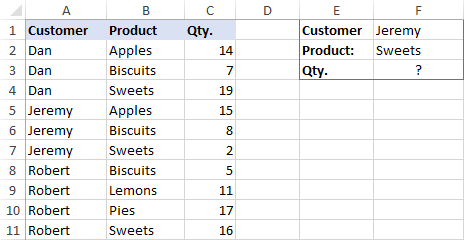A usual VLOOKUP formula won't work in this situation because it returns the first found match based on a single lookup value that you specify.

To overcome this, you can add a helper column and concatenate the values from two lookup columns (Customer and Product) there. It is important that the helper column should be the leftmost column in the table array because it's where Excel VLOOKUP always searches for the lookup value.

So, add a column to the left of your table and copy the below formula across that column. This will populate the helper column with the values from columns B and C (the space character is concatenated in between for better readability):

`=B2&" "&C2`

And then, use a standard VLOOKUP formula and place both criteria in the lookup_value argument, separated with a space:

`=VLOOKUP("Jeremy Sweets", A2:D11, 4, FALSE)`

Or, input the criteria in separate cells (G1 and G2 in our case) and concatenate those cells:

`=VLOOKUP(G1&" "&G2, A2:D11, 4, FALSE)`

As we want to return a value from column D, which is fourth in the table array, we use 4 for col_index_num. The range_lookup argument is set to FALSE to Vlookup an exact match. The screenshot below shows the result: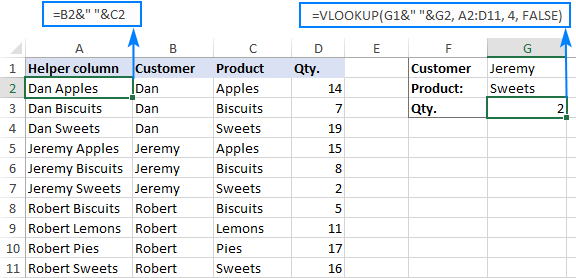In case your lookup table is in another sheet, include the sheet's name in your VLOOKUP formula. For example:

`=VLOOKUP(G1&" "&G2, Orders!A2:D11, 4, FALSE)`

Alternatively, create a named range for the lookup table (say, Orders) to make the formula easier-to-read:

`=VLOOKUP(G1&" "&G2, Orders, 4, FALSE)`

Note. For the formula to work correctly, the values in the helper column should be concatenated exactly the same way as in the lookup_value argument. For example, we used a space character to separate the criteria in both the helper column (B2&" "&C2) and VLOOKUP formula (G1&" "&G2).

### Formula 2. Excel VLOOKUP with multiple conditions

In theory, you can use the above approach to Vlookup more than two criteria. However, there are a couple of caveats. Firstly, a lookup value is limited to 255 characters, and secondly, the worksheet's design may not allow adding a helper column.

Luckily, Microsoft Excel often provides more than one way to do the same thing. To Vlookup multiple criteria, you can use either an INDEX MATCH combination or the XLOOKUP function recently introduced in Office 365.

For example, to look up based on 3 different values (Date, Customer name and Product), use one of the following formulas:

`=INDEX(D2:D11, MATCH(1, (G1=A2:A11) * (G2=B2:B11) * (G3=C2:C11), 0))`

`=XLOOKUP(1, (G1=A2:A11) * (G2=B2:B11) * (G3=C2:C11), D2:D11)`

Where:

• G1 is criteria 1 (date)
• G2 is criteria 2 (customer name)
• G3 is criteria 3 (product)
• A2:A11 is lookup range 1 (dates)
• B2:B11 is lookup range 2 (customer names)
• C2:C11 is lookup range 3 (products)
• D2:D11 is the return range (quantity)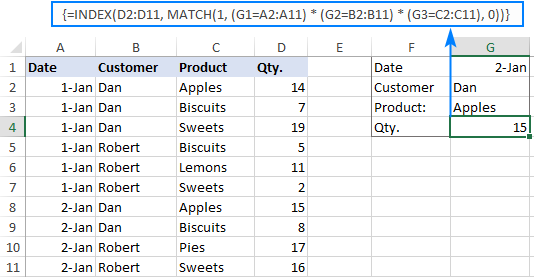Note. In all versions except Excel 365, INDEX MATCH should be entered as an CSE array formula by pressing Ctrl + Shift + Enter. In Excel 365 that supports dynamic arrays it also works as a regular formula.

For the detailed explanation of the formulas, please see:

## How to use VLOOKUP to get 2nd, 3rd or nth match

As you already know, Excel VLOOKUP can fetch only one matching value, more precisely, it returns the first found match. But what if there are several matches in your lookup array and you want to get the 2nd or 3rd instance? The task sounds quite intricate, but the solution does exist!

### Formula 1. Vlookup Nth instance

Suppose you have customer names in one column, the products they purchased in another, and you are looking to find the 2nd or 3rd product bought by a given customer.

The simplest way is to add a helper column to the left of the table like we did in the first example. But this time, we will populate it with customer names and occurrence numbers like "John Doe1", "John Doe2", etc.

To get the occurrence, use the COUNTIF function with a mixed range reference (the first reference is absolute and the second is relative like \$B\$2:B2). Since the relative reference changes based on a position of the cell where the formula is copied, in row 3 it will become \$B\$2:B3, in row 4 - \$B\$2:B4, and so on.

Concatenated with the customer name (B2), the formula takes this form:

`=B2&COUNTIF(\$B\$2:B2, B2)`

The above formula goes to A2, and then you copy it down to as many cells as needed.

After that, input the target name and occurrence number in separate cells (F1 and F2), and use the below formula to Vlookup a specific occurrence:

`=VLOOKUP(F1&F2, A2:C11, 3, FALSE)`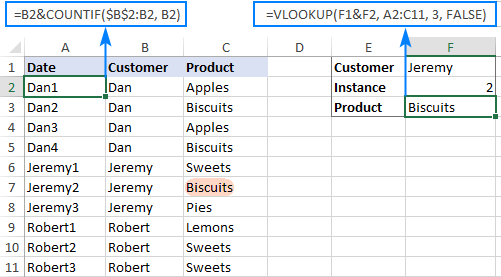### Formula 2. Vlookup 2nd occurrence

If you are looking for the 2nd instance of the lookup value, then you can do without the helper column. Instead, create the table array dynamically by using the INDIRECT function together with MATCH:

`=VLOOKUP(E1, INDIRECT("A"&(MATCH(E1, A2:A11, 0)+2)&":B11"), 2, FALSE)`

Where:

• E1 is the lookup value
• A2:A11 is the lookup range
• B11 is the last (bottom-right) cell of the lookup table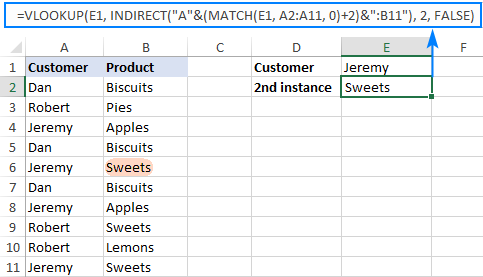Please note that the above formula is written for a specific case where data cells in the lookup table begin in row 2. If your table is somewhere in the middle of the sheet, use this universal formula, where A1 is the top-left cell of the lookup table containing a column header:

`=VLOOKUP(E1, INDIRECT("A"&(MATCH(E1, A2:A11, 0)+1+ROW(A1))&":B11"), 2, FALSE)`

#### How this formula works

Here is the key part of the formula that creates a dynamic vlookup range:

`INDIRECT("A"&(MATCH(E1, A2:A11, 0)+2)&":B11")`

The MATCH function configured for exact match (0 in the last argument) compares the target name (E1) against the list of names (A2:A11) and returns the position of the first found match, which is 3 in our case. This number is going to be used as the starting row coordinate for the vlookup range, so we add 2 to it (+1 to exclude the first instance and +1 to exclude row 1 with the column headers). Alternatively, you can use 1+ROW(A1) to calculate the necessary adjustment automatically based on the position of the header row (A1 in our case).

As the result, we get the following text string, which INDIRECT converts to a range reference:

`INDIRECT("A"&5&":B11") -> A5:B11`

This range goes to the table_array argument of VLOOKUP forcing it to start searching in row 5, leaving out the first instance of the lookup value:

`VLOOKUP(E1, A5:B11, 2, FALSE)`

## How to Vlookup and return multiple values in Excel

The Excel VLOOKUP function is designed to return just one match. Is there a way to Vlookup multiple instances? Yes, there is, though not an easy one. This requires a combined use of several functions such as INDEX, SMALL and ROW is an array formula.

For example, the below can find all occurrences of the lookup value F2 in the lookup range B2:B16 and return multiple matches from column C:

`{=IFERROR(INDEX(\$C\$2:\$C\$11, SMALL(IF(\$F\$1=\$B\$2:\$B\$11, ROW(\$C\$2:\$C\$11)-1,""), ROW()-1)),"")}`

There are 2 ways to enter the formula in your worksheet:

1. Type the formula in the first cell, press Ctrl + Shift + Enter, and then drag it down to a few more cells.
2. Select several adjacent cells in a single column (F1:F11 in the screenshot below), type the formula and press Ctrl + Shift + Enter to complete it.

Either way, the number of cells in which you enter the formula should be equal to or larger than the maximum number of possible matches.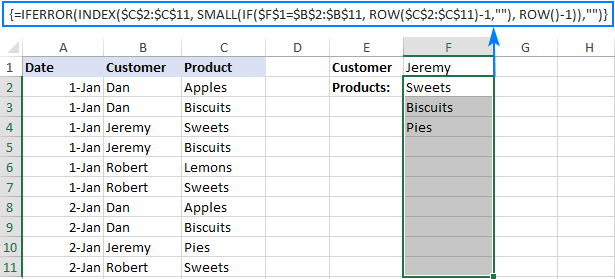For the detailed explanation of the formula logic and more examples, please see How to VLOOKUP multiple values in Excel.

## How to Vlookup in rows and columns (two-way lookup)

Two-way lookup (aka matrix lookup or 2-dimentional lookup) is a fancy word for looking up a value at the intersection of a certain row and column. There are a few different ways to do two-dimensional lookup in Excel, but since the focus of this tutorial is on the VLOOKUP function, we will naturally use it.

For this example, we'll take the below table with monthly sales and work out a VLOOKUP formula to retrieve the sales figure for a specific item in a given month.

With item names in A2:A9, month names in B1:F1, the target item in I1 and the target month in I2, the formula goes as follows:

`=VLOOKUP(I1, A2:F9, MATCH(I2, A1:F1, 0), FALSE)`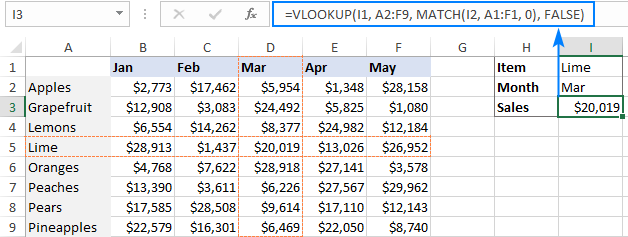### How this formula works

The core of the formula is the standard VLOOKUP function that searches for an exact match to the lookup value in I1. But since we do not know in which exactly column the sales for a specific month are, we cannot supply the column number directly to the col_index_num argument. To find that column, we use the following MATCH function:

`MATCH(I2, A1:F1, 0)`

Translated into English, the formula says: look up the I2 value in A1:F1 and return its relative position in the array. By supplying 0 to the 3rd argument, you instruct MATCH to find the value exactly equal to the lookup value (it's like using FALSE for the range_lookup argument of VLOOKUP).

Since Mar is in the 4th column in the lookup array, the MATCH function returns 4, which goes directly to the col_index_num argument of VLOOKUP:

`VLOOKUP(I1, A2:F9, 4, FALSE)`

Please pay attention that although the month names start in column B, we use A1:I1 for the lookup array. This is done in order for the number returned by MATCH to correspond to the column's position in table_array of VLOOKUP.

To learn more ways to perform matrix lookup in Excel, please see INDEX MATCH MATCH and other formulas for 2-dimensional lookup.

## How to do multiple Vlookup in Excel (nested Vlookup)

Sometimes it may happen that your main table and lookup table do not have a single column in common, which prevents you from doing a Vlookup between two tables. However, there exists another table, which does not contain the information you are looking for but has one common column with the main table and another common column with the lookup table.

In below image illustrates the situation: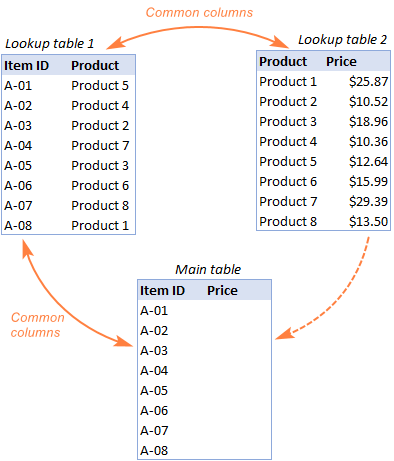The goal is to copy prices to the main table based on Item IDs. The problem is that the table containing prices does not have the Item IDs, meaning we will have to do two Vlookups in one formula.

For the sake of convenience, let's create a couple of named ranges first:

• Lookup table 1 is named Products (D3:E10)
• Lookup table 2 is named Prices (G3:H10)

The tables can be in the same or different worksheets.

And now, we will perform the so-called double Vlookup, aka nested Vlookup.

First, make a VLOOKUP formula to find the product name in the Lookup table 1 (named Products) based on the item id (A3):

`=VLOOKUP(A3, Products, 2, FALSE)`

Next, put the above formula in the lookup_value argument of another VLOOKUP function to pull prices from Lookup table 2 (named Prices) based on the product name returned by the nested VLOOKUP:

`=VLOOKUP(VLOOKUP(A3, Products, 2, FALSE), Prices, 2, FALSE)`

The screenshot below shows our nested Vlookup formula in action: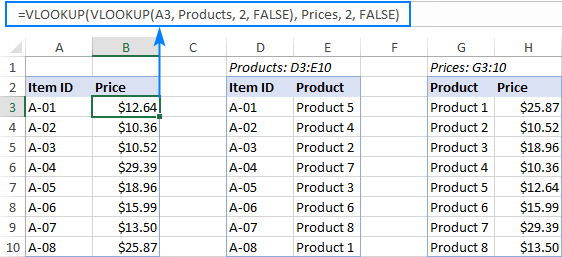## How to Vlookup multiple sheets dynamically

Sometimes, you may have data in the same format split over several worksheets. And your aim is to pull data from a specific sheet depending on the key value in a given cell.

This may be easier to understand from an example. Let's say, you have a few regional sales reports in the same format, and you are looking to get the sales figures for a specific product in certain regions: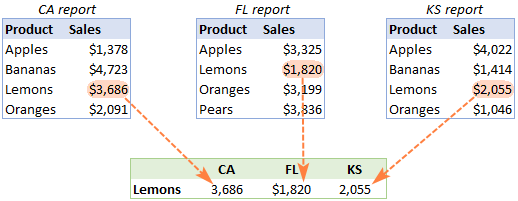Like in the previous example, we start with defining a few names:

• Range A2:B5 in CA sheet is named CA_Sales.
• Range A2:B5 in FL sheet is named FL_Sales.
• Range A2:B5 in KS sheet is named KS_Sales.

As you can see, all the named ranges have a common part (Sales) and unique parts (CA, FL, KS). Please be sure to name your ranges in a similar manner as it's essential for the formula we are going to build.

### Formula 1. INDIRECT VLOOKUP to dynamically pull data from different sheets

If your task is to retrieve data from multiple sheets, a VLOOKUP INDIRECT formula is the best solution – compact and easy-to-understand.

For this example, we organize the summary table in this way:

• Input the products of interest in A2 and A3. Those are our lookup values.
• Enter the unique parts of the named ranges in B1, C1 and D1.

And now, we concatenate the cell containing the unique part (B1) with the common part ("_Sales"), and feed the resulting string to INDIRECT:

`INDIRECT(B\$1&"_Sales")`

The INDIRECT function transforms the string into a name that Excel can understand, and you put it in the table_array argument of VLOOKUP:

`=VLOOKUP(\$A2, INDIRECT(B\$1&"_Sales"), 2, FALSE)`

The above formula goes to B2, and then you copy it down and to the right.

Please pay attention that, in the lookup value (\$A2), we've locked the column coordinate with absolute cell reference so that the column remains fixed when the formula is copied to the right. In the B\$1 reference, we locked the row because we want the column coordinate to change and supply an appropriate name part to INDIRECT depending on the column into which the formula is copied: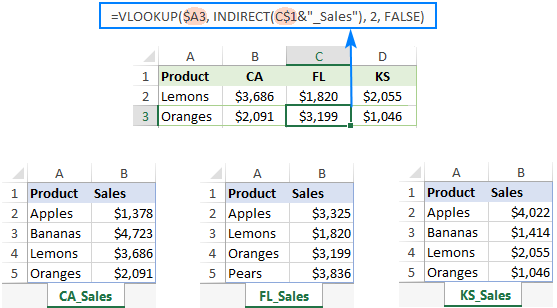If your main table is organized differently, the lookup values in a row and unique parts of the range names in a column, then you should lock the row coordinate in the lookup value (B\$1) and the column coordinate in the name parts (\$A2):

`=VLOOKUP(B\$1, INDIRECT(\$A2&"_Sales"), 2, FALSE)`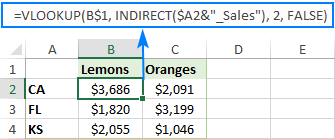### Formula 2. VLOOKUP and nested IFs to look up multiple sheets

In situation when you have just two or three lookup sheets, you can use a fairly simple VLOOKUP formula with nested IF functions to select the correct sheet based on the key value in a particular cell:

`=VLOOKUP(\$A2, IF(B\$1="CA", CA_Sales, IF(B\$1="FL", FL_Sales, IF(B\$1="KS", KS_Sales,""))), 2, FALSE)`

Where \$A2 is the lookup value (item name) and B\$1 is the key value (state):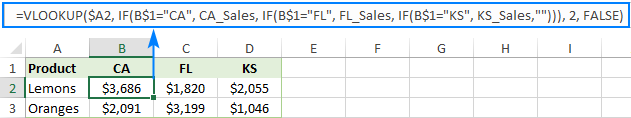In this case, you do not necessarily need to define names and can use external references to refer to another sheet or workbook.

For more formula examples, please see How to VLOOKUP across multiple sheets in Excel.

That's how to use VLOOKUP in Excel. I thank you for reading and hope to see you on our blog next week!

Advanced VLOOKUP formula examples (.xlsx file)

## You may also be interested in

1. Help Full

2. Hello Svetlana;

How I can adjust age brackets with vlookup formula using multiple criterias.

Plan Gender Age Contribution
Plan A Male 0-17 1,703
Plan A Female 0-17 1,703
Plan B Male 0-17 1,569
Plan B Female 0-17 1,569
Plan C Male 0-17 1,426
Plan C Female 0-17 1,426
Plan A Male 18-30 1,260
Plan A Female 18-30 1,264
Plan B Male 18-30 979
Plan B Female 18-30 979
Plan C Male 18-30 2,597
Plan C Female 18-30 3,866

I have the date in above mentioned form and I want contribution data on an other sheet using vlookup with other 3 criterias for exact match on other sheet.

3. Hi Svetlana
When doing the formula =IFERROR(VLOOKUP(\$F\$2,INDIRECT("\$F\$2,\$B\$2:\$B\$16,0)+2)&":\$C16"),2,FALSE),"")to identify the 2nd occurrence for each name with the appropriate product, my formula returns a 0 every time I change the name. This is despite copying the one from the download sample and changing any cell references to match above. Would have any ideas as to why this happens?. Thank you.

4. how to use H lookup and V lookup for archiving data any suitable example?

5. Thank you for this interesting post. I could not find what I was looking for however.

I need to find values in rows with multiples criteria.
In the example below I have the same material being ordered under several PO numbers.

I need a formula that will tell me how many pieces of material #2 have been ordered under PO #2

Data table
Material# PO# OnOrder

material1 PO1 5
material2 PO1 10
material3 PO1 15
material2 PO2 10
material3 PO2 8
material4 PO2 12

Result table

PO2 (citeria 1)
material2 (criteria 2) result = 10

Thanks

6. Hi, I'm doing my best to understand vlookups. I wanted to know if you can further break down the following formula that you had posted. I want to fully understand why it works. The formula is from the tutorial about looking up duplicates with vlookup. Thank you:

{=INDEX(\$C\$2:\$C\$16,SMALL(IF(\$F\$2=B2:B16,ROW(C2:C16)-1,""),ROW()-3))}

7. Hello,

I'm trying to add multiple VLOOKUP's together (week 1, 2, 3, etc), which I can do. But if one week is missing the item I'm looking up (person didn't take calls that week), it's giving me a "FALSE". I tried to use the ISERROR, but it keeps giving me a "0". I know why it gives the zero, but I'm not sure how to make it "skip" that week if the person isn't found.

Example:
IF(ISERROR((VLOOKUP(B2,'Team Stats Week 1'!B2:P21,2,FALSE))+(VLOOKUP(B2,'Team Stats Week 2'!B2:P21,2,FALSE))),0,(VLOOKUP(B2,'Team Stats Week 1'!B2:P21,2,FALSE))+(VLOOKUP(B2,'Team Stats Week 2'!B2:P21,2,FALSE)))

8. I am using google forms to make sure that staff read the circulars.
They fill up the form once they have read it.
The responses are automatically shown in a spreadsheet.

So, I have staff name in column B and Circular name in Column C
I want 0 to be shown in a column if a staff has read all 4 circulars.
How do I make that work?
Any help is greatly appreciated.

9. Hi Svetlana,
I want to fetch all items in comma separated in Sheet1, col(n) from Sheet 2 col(x) which look value is in col(x-2).

Please let me know how can i do this.

10. Hi Svetlana,

Thank you so much! Your explanation was perfect. I understand now how the formula works and was able to successfully complete my project. Thank you very much for the quick response!

-Will

11. Hi Svetlana,

This is one of the best technical Excel posts I have ever had the pleasure to read. Thank you and well done! I do have a question however, what precisely are the "Row" functions doing in the array formula? I am having a hard time unpacking what is going on there... I understand the syntax but not the context. Would you please break that down a little bit more?

Lastly, in your example: "How to get all duplicate values in the lookup range", how would you re-write the formula to report the purchased products next to the customer name, starting in cell G2 and continuing on to the right to cell J2, etc?

-Will

• Hi Will,

Thank you for your kind words and a great question : )

I thought other readers might want to know the details too, so I've added the formula explanation to the post, hopefully it will be helpful.

And here's the formula "to report the purchased products next to the customer name, starting in cell G2 and continuing on to the right to cell J2":

=IFERROR(INDEX(\$C\$2:\$C\$16, SMALL(IF(\$F\$2=\$B\$2:\$B\$16, ROW(\$C\$2:\$C\$16)-1,""), COLUMN()-6)),"")

12. i want to compare two spredsheet with name and amount in one sheet is contain in another sheet in same combination . wich formula i use

13. i want sheet to sheet multiply total formula .. excell sheet

• Hi Prem,

It is difficult to recommend anything based on such generic description. Most likely you need to use the SUMPRODUCT function.

14. This is great! Thank you

15. CAN WE GET MORE THAN ONE COLOUMN OF DATA SIMULTANEOUSLY BY VLOOKUP...???

16. Hi, I have a workbook with multiple tabs. In my master sheet I have values in column A (AA, BB, CC, DD, EE....for example). Then I have tabs labeled AA, BB, CC, DD, EE. I'm trying to do the same vlookup but on different tabs depending on what my master sheet column A value has...

So if Column A is BB I want the vlookup to look at sheet BB. Here is the manual way of doing it:

=VLOOKUP(B1,BB!A:B,2,FALSE)

Here is what i want the formula mimic so it works in a similar fashion:

=VLOOKUP(B2,A2&"!A:B",2,FALSE)

I also tried giving A:B on sheet BB a reference of 'BB' in hopes this would work:

=VLOOKUP(B2,A2,2,FALSE)

Any help is appreciated!!!

• I need to do the same sort of thing as Luke.

I Have a cell lets say its A1 that specifies from a drop-down list a Sheet Name (AA BB CC DD etc)
I want to pass that reference to a VLOOKUP Formula which would be SOMETHING LIKE =VLOOKUP(B5,A1&!D1:E22,2,False)
Where B5 is the cell containing theLookup_value, A1 is the cell containg the sheet name, and D1:E22 is the Table array.

I have been told the INDIRECT formula should work but I am jet to find a way to get a successful result.

Anyone Know?

17. I have the following
A 2
B 5
C 3

I want it to
A
A
B
B
B
B
B
C
C
C

Can you help me with excel formula

• Hello Daro,

A special VBA script will be the best solution to your task. Sorry, I cannot help with this.

18. I need a formula were in can get what ever the data i feed in from sheet 1 to sheet 2 in the same workbook ?

• Hello Samantha,

If you need to simply have the data copied from sheet1 to sheet2 as you enter it, you can put this formula in cell A1 on sheet2 and then copy it to all other cells:
=Sheet1!A1

19. Dear Sir,

Iam trying to do lookup with multiple cateria like

I have one sheet with account number,name & business

as well I have one sheet, when I will mentioned account number in sheet one formula will check aacount number & name then business will come

• Hello Sandeep,

A similar example is described in How to do a vlookup with multiple criteria. This example explains how to look up with 2 criteria "Customer Name" and "Product". Your can download the example in the end of that section and adjust the cell references in the formulas.

20. Dear Svetlana,

I am very much impressed by the guidelines you are providing for the problems faced by the Excel users. Really it is a quite best Website for having expert advice for advanced Excel users.

With regards.

CMA Anand

• Thank you very much for your kind words, Anand! I'm pleased to know you've found the tutorial helpful.

21. Dear Svetlana,

thank you for such a great site. I'm actually learning to use excel as more than just a list keeper. As I was using your formula for duplicate values in a look up range, I ran into a few problems. The information was only being picked up in one cell (I'm searching for dates). After I set the dates in the original column and the lookup column to the exact format, all of the dates show except for the first dates on the original list. I'm sure it's a small tweaky thing but I've been at it for 3 hours with no success. Do you have any suggestions on what I should look for as I'm troubleshooting? Thank you.

22. Hi Svetlana,
Thanks for the suggestions - there are some very good ones in here. I am familiar with the VLOOKUP formula, but have often wondered if there is a way to look through several worksheets. I have a roster template I use and I create a new worksheet for each roster. I would like a worksheet at the frontthat acts like a summary table, where the first column contains a code, which matches the name of the relevant roster worksheet. The rest of the columns in the table will find the appropriate worksheet (based on what is in the first column) and return the data from each field in my roster template.
Any tricks you could suggest would be appreciated.
Thanks.

23. I need help with a formula. Can someone assist?

24. I believe this is the info i've been looking for to put together this project that i've been trying to wrap my head around
What i would like to do is Input a "part#" using VLOOKUP to list all of the tools I need to make said part (i've accomplished this much) at the same time have it kick back a number that refers to current inventory for said tools (this number can change at any moment). So i know i'm going to need more than 1 spreadsheet to complete this task. I can run an inventory report anytime and export it to excel so my question is, can i build a template that i can drop my current inventory list in to that already has the formulas written out? What are the formulas i need to use to tie the 2 spreadsheets together? We have hundreds of different part numbers and thousands of tools and not having a system in place has bogged us down. End goal is to avoid "spot buying" and get ahead of the curve and forecast my tool ordering before the job hits the floor. Tool list's with the part #'s are already populated awaiting my completion of this task
Thank you much and i hope theres no confusion here.

• EXAMPLE
Input:866637
Return: 1/4 drill 4pcs
.3438 drill 2pcs
3/4 end mill 1pc
1/2-14 npsf 5pcs

i figured one workbook would be my part numbers with tools listed and the 2nd being my current inventory.

• Hello Russ,

I am sorry, it is difficult to recommend anything without seeing your data. If you can send a sample workbook to support@ablebits.com and include the result you want to get, our support team will try to help.

25. DATE CODE SUBJECT NAME TIME
10-Nov-14 EC-601 English - IV FN
10-Nov-14 M-601 English –IV FN
10-Nov-14 EC-304 Communication AN
10-Nov-14 M-307 Machine Drawing AN
11-Nov-14 EC-404 Microprocessors FN
11-Nov-14 M-401 English-III FN
11-Nov-14 EC-104 Engineering AN
11-Nov-14 M-107 Engineering Drawing AN
I want only one item in Code column to be displayed one by one at different locations based DATE and TIME columns

• Hello Aravind,

I am sorry, I cannot follow you. If you can send a sample workbook with your data to support@ablebits.com including the expected result, our support team will try to help.

26. Hi,
I need to get the PR number in Sheet: PO Short Table from Sheet : Pivot Table, based on Drawing ID and Material. Basically lookup & match 2 cells(A3,B3) and get the value from C3.
Which formula to use? Pls help.

Sheet : Pivot Table
Sum of PR Qty.
Drawing ID Material PR No. Total
LMV-41105060 SDSU16404121 3000053435 13

Sheet: PO Short Table
DWG ID System Matl No Matl Description PO Qty PR No
LMV-41105060 AFS SDSU16404121 STUD PAD 0

27. I am creating a new sales analysis file to track sales over several years. My file has multiple worksheets. The first sheet, Sheet1, has raw data downloaded from our order entry system. Column D in Sheet1 holds the invoice date.

Because we run on a Fiscal year cycle, not a calendar year cycle, I now want to automatically calculate the correct fiscal year for the invoice date into Sheet1, Column F.

I have created a table on Sheet2 which holds date ranges that corresponds to fiscal years, ie:
Sheet2, Column A, Rows 1 thru' 10 = StartDate
Sheet2, Column B, Rows 1 thru' 10 = End Date
Sheet2, Column C, Rows 1 thru' 10 = Fiscal Year

I have been struggling to find a formula that will automatically calculate this information for me on Sheet1 in Column F. I have tried index-match, vlookup, lookup, LessThan, etc and cannot get this figured out.

Any help would be greatly appreciated. Thank you.

• Hello Pam,

On Sheet 2, sort the dates by column A in ascending order, and then you can apply MATCH with the "less than" match_type parameter (1 or ommitted):
=INDEX(Sheet2!\$B\$1:\$B\$10,MATCH(D2, Sheet2!\$A\$1:\$A\$5,1))

• Svetlana - Thank you for the reply. I will definitely give that a try today! Your help is greatly appreciated. (Sorry for my slow reply back - I have been out of the office this past week with the 'flu and just came back today).

28. I have one issue with my formula to find the value from another table on the same sheet that you can see their example as below.

Table A Table B
A U V
1 TC10001 RQ12345 TC10003,TC10002
2 TC10002 RQ12346 TC10003
3 TC10003 RQ12347 TC10002,TC10001
. . . .
. . . .
. . . .
100 TC10100 RQ12445 TC10050,TC10003,TC10001

My formula is "=VLOOKUP("*"&MID(\$A1,3,5)&"*",U:V,1,FALSE)". Can you please help me to correct my formula? My expected result is formula should return at least only one value of column V.

Thanks a lot in advance for any help
Beer :)

29. Thanks for this helpful article; I use vlookup with match formula that has solved my problem.

Thanks again Deen.

30. {=IFERROR(INDEX(\$B\$2:\$B\$22,SMALL(IF(\$A\$24=A2:A22,ROW(B2:B22)-1,""),ROW()-3)),"")}

This formula isn't working for me, after i press Shft+cntrl+enter nothing comes up.

31. Thanks, You're really very helpful

32. Hello,

I have two sheets with data.In third sheet, I would like to display the value of one cell by checking it in two sheets simultaneously. Can we do this with vlook up.

Ex: Name Sales Name Sales Name Sales
A 2 D 5 B ?
B 6 E 10 E ?
C 8 F 4 D ?

As you can see there are two different tables with different values. There is third table with same names. Now I want to show there sales by looking into both the tables.

What is the formula that I should be looking.

Thanks,
Avinash B

33. I would like to copy this formula down multiple rows on a spreadsheet:
=IF(ISERROR(INDEX(\$A\$1:\$B\$8, SMALL(IF(\$A\$1:\$A\$8=\$E1, ROW(\$A\$1:\$A\$8)), ROW(1:1)),2)),"", INDEX(\$A\$1:\$B\$8, SMALL(IF(\$A\$1:\$A\$8=\$E1, ROW(\$A\$1:\$A\$8)), ROW(1:1)),2))

But my index and therefore row # needs to change according to when the index number changes. Let's say I have 8 skus in my assortment. I know the first 5 will be new skus, so I would have my item number (1234, 455, etc.) filled in, but the sku column is blank. The last three are skus I'm carrying over and I don't need to worry about them. When I use the formula above, it works for the first three items because it refers back to cell A1 which is the first 1234. But when I get to 455, it leaves a blank cell because it's looking for the 4th row of A1. Is there anyway to have it move to the new number of 455 without having to retype in the new row number each time?

1234 this is new sku
1234 so is this
1234 and this
455 new sku1
455 new sku2
819 old sku1 - no formula
819 old sku2 - no formula
222 filled in - no formula

34. i have partial number like 456 out of full value 123456789(under id heading)
here table contains id,product,customer,q1,q2,a3

so how can i retrieve the full value(123456789) with the help of 456

35. Hello Svetlana,

Thanks for this tutorial. Your handling of Excel functions is too good.

36. I have a data of around 40000 rows of inter unit transfer entries and i have to get prices or data from other sales near to that date or before that date maximum 30 to 40 days.with the help of concatenate formula i made a key to get the data with the help of vlookup but the issue faced by me the vlookup function pick the value which it found first in the data but i have to get data near by to that date or of the the same date.I'm not understanding which formula we can use to extract data from the normal sales.Because the data is to huge it is very difficult to do this manually.IF it is possible please tell me the solution.

37. I am looking for a formula of vlookup in which we can use concatenate key of various columns and that is used on the basis of other date function like i have to select only that data that is nearby to that or below that date and the concatenated key may contain various similar entries but on different date.Have you any idea how can i do this.i want to this for transfer prices because it contains a huge data.

38. I am looking to do a lookup on based on two criteria, where one of the criteria would be based on a range rather than an exact match. Do you know how I can do that?

Here are the 2 criteria:
Lumen
Output Code
2170 32.F
4970 32.F
4971 42.CF
1185 15.CF
1407 32.F
1185 32.F
1086 32.F

The codes are an exact match, but the lumen output would fall between the following ranges, so for example, I'd want 2170 to return 18. I used the following formula to find a match based on the 2 criteria but it only works if it's exact: =LOOKUP(2,1/(B3:B13=C16)/(D3:D13=C18),(C3:C13))

Lumen
Output Wattage
1350 9
1800 12
1800 12
2100 14
2700 18
3300 22
3300 22
4970 28
5400 36
6600 44

Andrea

• Hello Andrea,

For Lumens, you can use the Match function with of "greater than" match_type (the 3rd argument is -1). So, first off sort your table from largest to smallest, and then use this formula:
=INDEX(B2:B11,MATCH(2170,A2:A11,-1))

Where A2:B11 is the table with your data.

If you are looking for something different, you can send us your workbook at suport@ablebits.com and include the result you are looking for.

• Thank you so much. And I can use this formula to match multiple criteria:

{=INDEX(C3:C13,MATCH(1,(B3:B13=C16)*(D3:D13=C18)*(E3:E13=C18),0))}

39. Hi,
I am really struggling on a work data base that I am trying to set up…
We start of with the Master Orders Workbook which consists of a summery sheet and store order sheets. We have to copy and past the order that come in onto this workbook.
I have then set up a Master POD’s workbook which pulls through all of the information put on to the Master Orders….but as this goes to our courier company they only need to see certain columns. This is all ok and working.
I am now stuck, as when the original orders come through there are 2 different warehouses on it that we pick from. WH1 in Singapore and WH2 in the UK my issue is that at the moment we have to manually go through all of the orders and split them into WH1 and WH2.
Is there a way of setting up a Master WH1 workbook were it will only pull this information through, even though the info I need it to look at is in column J and once it has found this I also need it to pull through the corresponding rows. I can’t provide the sum with an exact table as the size as the orders change for each order placed.
I am hoping that once I have sorted this out I will be able to do the same for WH2 and also cut my work load down by a lot!!
If you have any ideas it would be much appreciated.
Many thank, Fran

• Hello Fran,

I am sorry, it is difficult to suggest anything without seeing your data. If you can send a sample workbook and the detailed description of workflow to support@ablebits.com, we'll try to help you.

40. Hi!
I'm trying to use your formula which I have put together as follow:
=IFERROR(INDEX(RFVDTL!\$F\$2:\$F\$1757, SMALL(IF(\$D\$2=RFVDTL!A2:A1757,ROW(RFVDTL!F2:F1757)-1,""), ROW()-3)),"")

However, when I press Ctrl + Shift + Enter, I get an error s which is "Array Formulas are not valid in merged cell" can you help please?

• Hi!

The point is that you are trying to apply an array formula to merged cells, which is not possible.

You can either unmerge the cells or enter an array formula into any other non-merged cell. If you choose the latter, then type =X20 in your merged cell, where X20 is the cell that contains an array formula.

41. I am trying to get all duplicate values in the lookup range, but I need help because some of the names on the left only contain part of the name. For example, I want to find all the part numbers for any "gold" material. So the names on the left could be:

Gold rock 123
Solid gold chair 234
Silver and gold frame 567

So could I put in "gold", and have it produce all three of those part numbers? instead of an exact match? I hope this makes sense.

Thanks

• Hello Kat,

You need to add a helper column to your source table and enter the following formula there:
=IF(ISERR(SEARCH("gold",A2))=FALSE,"OK","")

Where A is the column with the original text.

Then in the master table, search for all "OK" instances in your helper column using formulas and pull out the corresponding Part numbers.

42. With this formula in F4 -- {=IFERROR(INDEX(\$C\$2:\$C\$16,SMALL(IF(\$F\$2=B2:B16,ROW(C2:C16)-1,""),ROW()-3)),"")}
I get "Apples".

How do I get the information on the remaining rows to show up in F5 etc..

Thanks in advance for any help.

• Hello Ann,

Simply copy the below formula down to a few more cells, e.g. F4:F8, as in the described example. The number of cells where you copy the formula should be equal to or larger than the maximum number of entries the formula may return.

43. can you help me work out this formula

I need to search number that can be more than 1 = SHEET 2
then if it is more that 1 word, I need to add them

have the number in SHEET 1 = need the output "apple / orange / grapes" for 293
search the number and fruits in SHEET 2

example
293 apple
293 orange
293 grapes
294 mango

need output "apple / orange / grapes" for 293
need output "mango / avocado" for 293

44. Hi,

I'm hoping you can point me in the right direction for a project I'm working on.

I have a table or list of values which I need to search for in an excel worksheet. So for example, the list would be hammer, nails, screws, bricks, etc. And the text would be...in cell A1 "You should have 25 bricks, 10 penny nails and a rubber hammer to complete the project." In cell A2, "Begin by laying out the bricks"...So what I need to do is to write a macro to go through the list, item by item and COUNTIF I get a hit within a range of text, A1:D45. In this case bricks are mentioned 2x, nails 1x and hammer 1x. Also, the length of both the incoming list and the text I'm looking through will be variable. Does that make sense?

Many thanks,

• Hi Bonnie,

In theory, you can fulfill this task using formulas, but a more flexible and quick way would be to use a VBA macro. You can search for it in special VBA sections on these forums: excelforum and mrexcel.com.

• I am having a sheet with names in one column, and in another sheet with names and numbers. i have used vlookup to get the number from sheet 2 to appropriate value in sheet 1. The difficulty i am facing is in sheet 2 same names are there for different numbers, so vlookup is giving the first match value and leaving the rest. Help me to solve this issue.
like for the name glass there may be 100 101 102 456..numbers, if i am comparing glass from sheet 1 to sheet 2 it is just picking 100 for all the rest of the names (having glass in sheet 1).

• Hello Sai,

Please have a look at the Get 2nd, 3rd occurrence of the lookup value example. If you want to get something different, please describe the expected result in more detail.

45. Hi Svetlana, I am facing issue in V lookup, as I want the 2nd 3rd or 4th lookup value in a different tab but also I dont want to add a helper column. Can you please suggest how could I do that? Your help is appreciated.

• Hi Roshan,

For the 2nd lookup you can use the following formula (described in the article):
=IFERROR(VLOOKUP(\$F\$2,INDIRECT("\$B\$"&(MATCH(\$F\$2,Table4[Customer Name],0)+2)&":\$C16"),2,FALSE),"")

You can modify it for the 3rd and 4th lookups, but these will be very long, complicated and slow formulas. That's why I'd rather go with a helper column and then hide it.

46. Hi Svetlana,

This is the first time, i have visited your articles. And i am feeling that why did not i come across this quite earlier...!! Awesome ideas..!!

Thanks

47. Thank you for your articles...thanks...

48. Thank you Svetlana for this article. A lot of good ideas there.
:)

49. Hi

I have a roster spreadsheet i need to use the vlookup function to calculate the hours delivered to each client i tried to follow your formula didn't work for me can you help ?

Thanks

• Hi Daniel,

I need to know more details about your data to be able to suggest a proper formula. If you can send me your sample workbook at support@ablebits.com along with the result you want to achieve, I will try to help.

50. Please fix the section title:

Use VLOOKUP and INDERECT to dynamically pull data from different sheets

It should be INDIRECT

I respect your site very much. It's just that spelling errors are a pet peeve of mine.

• Hi Rick,

Thank you very much for spotting this error, fixed! My spell checker ignores capitalized words, and here it is : (

• Very useful site, was looking over a formula from past 2 - 3 days, finally got it here with possible result which i was expecting for. Will surely mail you if any help required in future